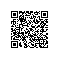深度学习高能干货：手把手教你搭建MXNet框架

01 NDArray

NDArray是MXNet框架中数据流的基础结构，NDArray的官方文档地址是：

https://mxnet.apache.org/api/python/ndarray/ndarray.html

http://www.numpy.org/

import mxnet as mx
import numpy as np
a = mx.nd.array([[1,2],[3,4]])
print(a)

[[1. 2.]
[3. 4.]]
<NDArray 2x2 @cpu(0)>

b = np.array([[1,2],[3,4]])
print(b)

[[1 2]
[3 4]]

print(a.shape)

(2, 2)

print(b.shape)

(2, 2)

print(a.dtype)

<class 'numpy.float32'>

print(b.dtype)

int64

c=mx.nd.array([[1,2],[3,4]], dtype=np.int8)
print(c.dtype)

<class 'numpy.int8'>

d = np.array([[1,2],[3,4]], dtype=np.int8)
print(d.dtype)

int8

c = mx.nd.array([[1,2,3,4],[5,6,7,8]])
print(c[0,1:3])

[2. 3.]
<NDArray 2 @cpu(0)>

d = np.array([[1,2,3,4],[5,6,7,8]])
print(d[0,1:3])

[2 3]

print(c)

[[1. 2. 3. 4.]
[5. 6. 7. 8.]]
<NDArray 2x4 @cpu(0)>

f = c.copy()
print(f)

[[1. 2. 3. 4.]
[5. 6. 7. 8.]]
<NDArray 2x4 @cpu(0)>

f[0,0] = -1
print(f)

[[-1. 2. 3. 4.]
[ 5. 6. 7. 8.]]
<NDArray 2x4 @cpu(0)>

print(c)

[[1. 2. 3. 4.]
[5. 6. 7. 8.]]
<NDArray 2x4 @cpu(0)>

e = c
print(e)

[[1. 2. 3. 4.]
[5. 6. 7. 8.]]
<NDArray 2x4 @cpu(0)>

e[0,0] = -1
print(e)

[[-1. 2. 3. 4.]
[ 5. 6. 7. 8.]]
<NDArray 2x4 @cpu(0)>

print(c)

[[-1. 2. 3. 4.]
[ 5. 6. 7. 8.]]
<NDArray 2x4 @cpu(0)>

g=e.asnumpy()
print(g)

[[-1. 2. 3. 4.]
[ 5. 6. 7. 8.]]

NumPy array转NDArray可以通过mxnet.ndarray.array()接口来实现：

print(mx.nd.array(g))

[[-1. 2. 3. 4.]
[ 5. 6. 7. 8.]]
<NDArray 2x4 @cpu(0)>?

print(e.context)

cpu(0)

e = e.as_in_context(mx.gpu(0))
print(e.context)

gpu(0)

MXNet框架中NDArray对象的默认初始化环境是CPU，在不同的环境中，变量初始化其实就是变量的存储位置不同，而且存储在不同环境中的变量是不能进行计算的，比如一个初始化在CPU中的NDArray对象和一个初始化在GPU中的NDArray对象在执行计算时会报错：

f = mx.nd.array([[2,3,4,5],[6,7,8,9]])
print(e+f)

mxnet.base.MXNetError: [11:14:13] src/imperative/./imperative_utils.h:56: Check failed: inputs[i]->ctx().dev_mask() == ctx.dev_mask() (1 vs. 2) Operator broadcast_add require all inputs live on the same context. But the first argument is on gpu(0) while the 2-th argument is on cpu(0)

f = f.as_in_context(mx.gpu(0))
print(e+f)

[[  1.   5.   7.   9.]
[ 11.  13.  15.  17.]]
<NDArray 2x4 @gpu(0)>

NDArray是MXNet框架中使用最频繁也是最基础的数据结构，是可以在CPU或GPU上执行命令式操作（imperative operation）的多维矩阵，这种命令式操作直观且灵活，是MXNet框架的特色之一。因为在使用MXNet框架训练模型时，几乎所有的数据流都是通过NDArray数据结构实现的，因此熟悉该数据结构非常重要。

02 Symbol

Symbol是MXNet框架中用于构建网络层的模块，Symbol的官方文档地址是：

https://mxnet.apache.org/api/python/symbol/symbol.html

import mxnet as mx
data = mx.sym.Variable('data')
conv = mx.sym.Convolution(data=data, num_filter=128, kernel=(3,3), pad=(1,1), name='conv1')
bn = mx.sym.BatchNorm(data=conv, name='bn1')
relu = mx.sym.Activation(data=bn, act_type='relu', name='relu1')
pool = mx.sym.Pooling(data=relu, kernel=(2,2), stride=(2,2), pool_type='max', name='pool1')
fc = mx.sym.FullyConnected (data=pool, num_hidden=2, name='fc1')
sym = mx.sym.SoftmaxOutput (data=fc, name='softmax')

mx.sym是mxnet.symbol常用的缩写形式，后续篇章默认采用这种缩写形式。另外在定义每一个网络层的时候最好都能指定名称（name）参数，这样代码看起来会更加清晰。

print(sym.list_arguments())

['data', 'conv1_weight', 'conv1_bias', 'bn1_gamma', 'bn1_beta', 'fc1_weight', 'fc1_bias', 'softmax_label']

arg_shape,out_shape,aux_shape = sym.infer_shape(data=(1,3,10,10))
print(arg_shape)
print(out_shape)
print(aux_shape)

[(1, 3, 10, 10), (128, 3, 3, 3), (128,), (128,), (128,), (2, 3200), (2,), (1,)]
[(1, 2)]
[(128,), (128,)]

sym_mini = sym.get_internals()['pool1_output']
print(sym_mini.list_arguments())

['data', 'conv1_weight', 'conv1_bias', 'bn1_gamma', 'bn1_beta']

fc_new = mx.sym.FullyConnected (data=sym_mini, num_hidden=5, name='fc_new')
sym_new = mx.sym.SoftmaxOutput (data=fc_new, name='softmax')
print(sym_new.list_arguments())

['data', 'conv1_weight', 'conv1_bias', 'bn1_gamma', 'bn1_beta', 'fc_new_weight', 'fc_new_bias', 'softmax_label']

import mxnet as mx
data_a = mx.sym.Variable ('data_a')
data_b = mx.sym.Variable ('data_b')
data_c = mx.sym.Variable ('data_c')
s = data_c*(data_a+data_b)
print(type(s))

<class 'mxnet.symbol.symbol.Symbol'>

e = s.bind(mx.cpu(), {'data_a':mx.nd.array([1,2,3]), 'data_b':mx.nd.array([4,5,6]),

'data_c':mx.nd.array([2,3,4])})

print(type(e))

<class 'mxnet.executor.Executor'>

output=e.forward()
print(output)

[ 10. 21. 36.]
<NDArray 3 @cpu(0)>

import mxnet as mx
data_a = mx.nd.array([1,2,3])
data_b = mx.nd.array([4,5,6])
data_c = mx.nd.array([2,3,4])
result = data_c*(data_a+data_b)
print(result)

[ 10. 21. 36.]
<NDArray 3 @cpu(0)>

data = mx.nd.arange(0,28).reshape((1,1,4,7))
print(data)

[[[[ 0.  1.  2.  3.  4.  5.  6.]
[ 7.  8.  9. 10. 11. 12. 13.]
[14. 15. 16. 17. 18. 19. 20.]
[21. 22. 23. 24. 25. 26. 27.]]]]
<NDArray 1x1x4x7 @cpu(0)>

weight和bias就是卷积层的参数值，为了简单起见，这里将weight初始化成值全为1的4维变量，bias初始化成值全为0的1维变量，这样就能得到最后的卷积结果。具体代码如下：

conv1 = mx.nd.Convolution(data=data, weight=mx.nd.ones((10,1,3,3)),
bias=mx.nd.zeros((10)), num_filter=10, kernel=(3,3),
name='conv1')
print(conv1)

[[[[ 72.  81.  90.  99. 108.]
[135. 144. 153. 162. 171.]]
[[ 72.  81.  90.  99. 108.]
[135. 144. 153. 162. 171.]
[[ 72.  81.  90.  99. 108.]
[135. 144. 153. 162. 171.]]
[[ 72.  81.  90.  99. 108.]
[135. 144. 153. 162. 171.]]
[[ 72.  81.  90.  99. 108.]
[135. 144. 153. 162. 171.]]
[[ 72.  81.  90.  99. 108.]
[135. 144. 153. 162. 171.]]
[[ 72.  81.  90.  99. 108.]
[135. 144. 153. 162. 171.]]
[[ 72.  81.  90.  99. 108.]
[135. 144. 153. 162. 171.]]
[[ 72.  81.  90.  99. 108.]
[135. 144. 153. 162. 171.]]
[[ 72.  81.  90.  99. 108.]
[135. 144. 153. 162. 171.]]]]
<NDArray 1x10x2x5 @cpu(0)>

03 Module

https://mxnet.apache.org/api/python/module/module.html

Module接口提供了许多非常方便的方法用于模型训练，只需要将准备好的数据、超参数等传给对应的方法就能启动训练。

bind操作中还存在一个重要的参数是for_training，这个参数默认是True，表示接下来要进行的是训练过程，因为我们这里只需要进行网络的前向计算操作，因此将该参数设置为False。

mod = mx.mod.Module(symbol=sym, context=mx.gpu(0))
mod.bind(data_shapes=[('data',(8,3,28,28))],
label_shapes=[('softmax_label',(8,))],
for_training=False)
mod.init_params()

data = mx.nd.random.uniform(0,1,shape=(8,3,28,28))
mod.forward(mx.io.DataBatch([data]))
print(mod.get_outputs())

[[ 0.50080067  0.4991993 ]
[ 0.50148612  0.49851385]
[ 0.50103837  0.4989616 ]
[ 0.50171131  0.49828872]
[ 0.50254387  0.4974561 ]
[ 0.50104254  0.49895743]
[ 0.50223148  0.49776852]
[ 0.49780959  0.50219035]]
<NDArray 8x2 @gpu(0)>

https://github.com/miraclewkf/MXNet-Deep-Learning-in-Action

import mxnet as mx
import logging

data = mx.sym.Variable('data')
conv = mx.sym.Convolution(data=data, num_filter=128, kernel=(3,3), pad=(1,1),
name='conv1')
bn = mx.sym.BatchNorm(data=conv, name='bn1')
relu = mx.sym.Activation(data=bn, act_type='relu', name='relu1')
pool = mx.sym.Pooling(data=relu, kernel=(2,2), stride=(2,2), pool_type='max',
name='pool1')
fc = mx.sym.FullyConnected(data=pool, num_hidden=2, name='fc1')
sym = mx.sym.SoftmaxOutput(data=fc, name='softmax')

data = mx.nd.random.uniform(0,1,shape=(1000,3,224,224))
label = mx.nd.round(mx.nd.random.uniform(0,1,shape=(1000)))
train_data = mx.io.NDArrayIter(data={'data':data},
label={'softmax_label':label},
batch_size=8,
shuffle=True)

print(train_data.provide_data)
print(train_data.provide_label)
mod = mx.mod.Module(symbol=sym,context=mx.gpu(0))
mod.bind(data_shapes=train_data.provide_data,
label_shapes=train_data.provide_label)
mod.init_params()
mod.init_optimizer()
eval_metric = mx.metric.create('acc')
for epoch in range(5):
end_of_batch = False
eval_metric.reset()
data_iter = iter(train_data)
next_data_batch = next(data_iter)
while not end_of_batch:
data_batch = next_data_batch
mod.forward(data_batch)
mod.backward()
mod.update()
mod.update_metric(eval_metric, labels=data_batch.label)
try:
next_data_batch = next(data_iter)
mod.prepare(next_data_batch)
except StopIteration:
end_of_batch = True
eval_name_vals = eval_metric.get_name_value()
print("Epoch:{} Train_Acc:{:.4f}".format(epoch, eval_name_vals))
arg_params, aux_params = mod.get_params()
mod.set_params(arg_params, aux_params)
train_data.reset()

logger = logging.getLogger()
logger.setLevel(logging.INFO)
mod.fit(train_data=train_data, num_epoch=5)

import mxnet as mx
import logging

data = mx.sym.Variable('data')
conv = mx.sym.Convolution(data=data, num_filter=128, kernel=(3,3), pad=(1,1),
name='conv1')
bn = mx.sym.BatchNorm(data=conv, name='bn1')
relu = mx.sym.Activation(data=bn, act_type='relu', name='relu1')
pool = mx.sym.Pooling(data=relu, kernel=(2,2), stride=(2,2), pool_type='max',
name='pool1')
fc = mx.sym.FullyConnected(data=pool, num_hidden=2, name='fc1')
sym = mx.sym.SoftmaxOutput(data=fc, name='softmax')

data = mx.nd.random.uniform(0,1,shape=(1000,3,224,224))
label = mx.nd.round(mx.nd.random.uniform(0,1,shape=(1000)))
train_data = mx.io.NDArrayIter(data={'data':data},
label={'softmax_label':label},
batch_size=8,
shuffle=True)

print(train_data.provide_data)
print(train_data.provide_label)
mod = mx.mod.Module(symbol=sym,context=mx.gpu(0))

logger = logging.getLogger()
logger.setLevel(logging.INFO)
mod.fit(train_data=train_data, num_epoch=5)

INFO:root:Epoch Train-accuracy=0.515000
INFO:root:Epoch Time cost=4.618
INFO:root:Epoch Train-accuracy=0.700000
INFO:root:Epoch Time cost=4.425
INFO:root:Epoch Train-accuracy=0.969000
INFO:root:Epoch Time cost=4.428
INFO:root:Epoch Train-accuracy=0.988000
INFO:root:Epoch Time cost=4.410
INFO:root:Epoch Train-accuracy=0.999000
INFO:root:Epoch Time cost=4.425

04 小结

NDArray是MXNet框架中最基础的数据结构，借鉴了NumPy中array的思想且能在GPU上运行，同时采取命令式编程的NDArray在代码调试上非常灵活。NDArray提供了与NumPy array相似的方法及属性，因此熟悉NumPy array的用户应该能够很快上手NDArray的操作，而且二者之间的转换也非常方便。

Symbol是MXNet框架中定义网络结构层的接口，采取符号式编程的Symbol通过构建静态计算图可以大大提高模型训练的效率。Symbol中提供了多种方法用于查看Symbol对象的信息，包括参数层、参数维度等，同时也便于用户在设计网络结构的过程中查漏补缺。

Module是MXNet框架中封装了训练模型所需的大部分操作的高级接口，用户可以通过Module模块执行bind操作、参数初始化、优化器初始化、模型的前向计算、损失函数的反向传播、网络参数更新、评价指标计算等，同时，Module模块还将常用的训练操作封装在了fit()方法中，通过该方法，用户可以更加方便地训练模型，可以说是既灵活又简便。